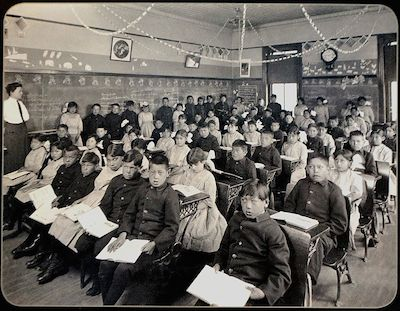# Probability of real life situations

Probability Level 5In competitive examinations that are given by a large number of people, (for example the JEE MAINS) in which many people getting the same score on the examination, there often arises a need for further ranking of the students. Usually the next data that is checked is their marks in a particular section.

Let's find the probability that two people are tied in their total marks, and also in the most important section. In the JEE, the most important section is Maths, followed by Physics and Chemistry.

The exam is has a total of 30 marks, with 3 sections namely physics, chemistry, maths, each worth 10 marks. Candidates A and B are sitting for this exam. Assume that in each section, their score is uniformly distributed over the integers 0 to 10 (inclusive).

The probability that A and B get the same total marks as well as same marks in the maths section is given by:

$P={ \left(\dfrac { a }{ b } \right) }^{ c }$

Find $a+b+c$

Details and Assumptions:

• $a,b,c$ are positive integers with $1 and $\gcd(a,b)=1$

• We have no idea of the intelligence of A and B hence we make the simplifying assumption that the total marks as well the marks they get in each section is totally random, i.e. they are equally likely to score 0, 5, or 10.

×